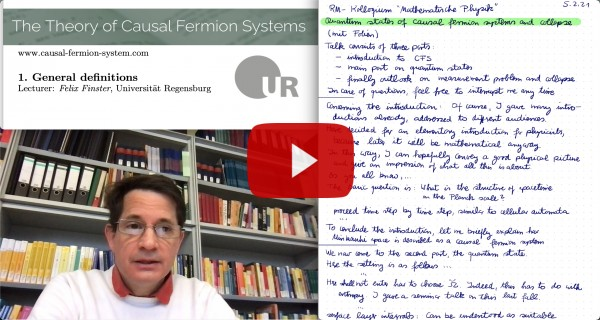# The Theory of Causal Fermion Systems

## Geometric Structures## The Spin Connection

The kernel of the fermionic projector $P(x,y) : S_y \rightarrow S_x$ relates different spacetime points to each other. In [lqg11] it is shown that, using a polar decomposition of $P(x,y)$, one can construct a unitary mapping $D_{x,y} \::\: S_y \rightarrow S_x$, referred to as the spin connection. More precisely, the spin connection has the form

$D_{x,y} = e^{i \varphi_{xy}\, v_{xy}}\: A_{xy}^{-\frac{1}{2}}\: P(x,y) \:,$

where $A_{xy}:= P(x,y)\, P(y,x) : S_x \rightarrow S_x$ is the closed chain, $v_{xy}$  is the directional sign operator, and $\varphi_{xy}$ is a suitably chosen real number. The conditions needed for the spin connection to be well-defined are subsumed in the notion that $x$ and $y$ must be spin connectable.

## Curvature

Curvature is defined via the holonomy of the spin connection,

$\mathfrak{R}(x,y,z) = D_{x,y}\:D_{y,z}\: D_{z,x} \::\: S_x \longrightarrow S_x \:.$

In [lqg11] the following inherent structures are defined for a general causal fermion system:

• Clifford subspaces and Clifford multiplication
• tangent space
• a metric connection $\nabla_{x,y}$, which is shown to be compatible with Clifford multiplication and the spin connection.

Moreover, in [lqg11] it is shown that in a specific limiting case, these objects go over to the corresponding objects of spin geometry in a globally hyperbolic spacetime.

For more details we also refer to the introduction and survey in [nrstg17] and to the talk on geometric structures at the 2018 spring school on causal fermion systems.